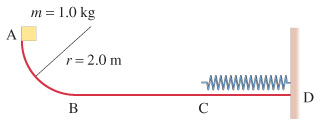# Problem: Consider the track shown in the figure. The section AB is one quadrant of a circle of radius 2.0 m and is frictionless. B to C is a horizontal span 3.1 m long with a coefficient of kinetic friction exttip{mu_{ m k}}{mu} = 0.24. The section CD under the spring is frictionless. A block of mass 1.0 kg is released from rest at A. After sliding on the track, it compresses the spring by 0.30 m . Determine the velocity of the block at point B.Determine the thermal energy produced as the block slides from B to C.Determine the velocity of the block at point C.Determine the stiffness constant k for the spring.

⚠️Our tutors found the solution shown to be helpful for the problem you're searching for. We don't have the exact solution yet.

###### Problem Details

Consider the track shown in the figure. The section AB is one quadrant of a circle of radius 2.0 m and is frictionless. B to C is a horizontal span 3.1 m long with a coefficient of kinetic friction = 0.24. The section CD under the spring is frictionless. A block of mass 1.0 kg is released from rest at A. After sliding on the track, it compresses the spring by 0.30 m .Determine the velocity of the block at point B.

Determine the thermal energy produced as the block slides from B to C.

Determine the velocity of the block at point C.

Determine the stiffness constant k for the spring.